# Make LEFT Function recognize a number in Excel

Are you wondering if there is a way to force a LEFT function to recognize a number?

Let see how we use Left function in Excel?

The LEFT function treats numbers (digits) as Text, evenwhen contained in a true Number entry. Therefore, when using digits as operators within these functions, the output must be treated as Text.

We will use the LEFT & VALUE functions to make a formula that will convert text into numbers.

LEFT: Returns the specified number of characters from the start of a text string.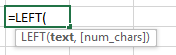Text:It is the text string from which you need to extract the characters.

num_chars:It specifies the number of characters from the left which you want to extract.

VALUE: Converts a text string that represents a number to a number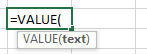Text:It is the text value which needs to be converted into numbers

Let us take an example to see that how we can use Left formula in Excel:

• We have some telephone numbers in column A
• We need to find the first 3 numbers of these phone numbers.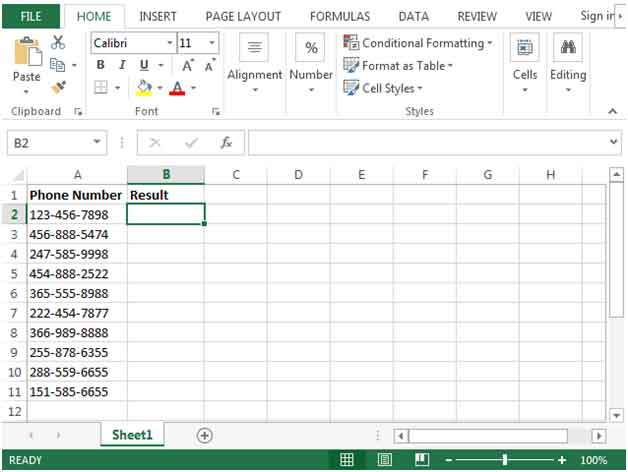• In cell B2,enter the formula =VALUE(LEFT(A2,3))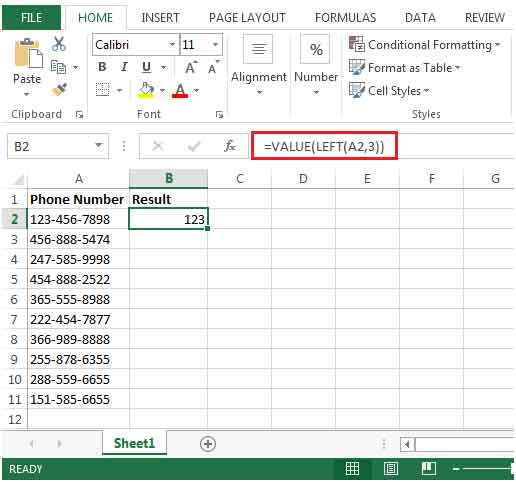• The LEFT function will extract the first 3 digits but will be displayed as text (left aligned) &the VALUE function will convert the output into numbers (right aligned).
• We can copy the formula down to the range B3:B11 to achieve the desired result for the whole data set.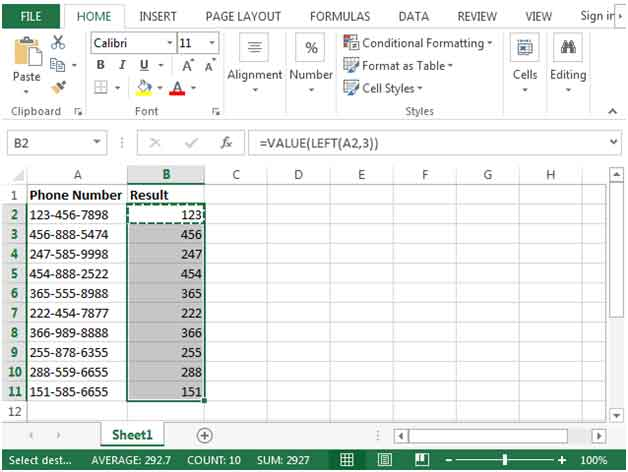We would love to hear from you, do let us know how we can improve, complement or innovate our work and make it better for you. Write us at info@exceltip.com

## Users are saying about us...

1.How to separate number from this sentence ajdjfjdjfjfjf 123345 sjdjdhfhf

Terms and Conditions of use

The applications/code on this site are distributed as is and without warranties or liability. In no event shall the owner of the copyrights, or the authors of the applications/code be liable for any loss of profit, any problems or any damage resulting from the use or evaluation of the applications/code.